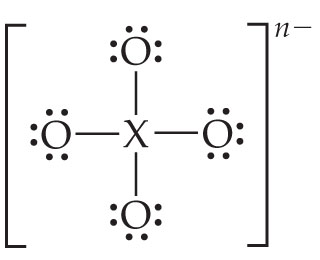# Problem: Consider the Lewis structure for the polyatomic oxyanion shown here, where X is an element from the third period (Na-Ar). By changing the overall charge, n, from 1– to 2– to 3– we get three different polyatomic ions.For each of these ions determine the formal charge of the central atom, X. Arrange your answers in order increasing n.

###### FREE Expert Solution

Recall: Formal charge is given by:

F.C. = # of val. e  – (# of bonds + # of lone pair e)

Based on the given Lewis structure, there are a total 32 valence electrons. We can use this information to determine the number of valence electrons of the unknown element.

For the polyatomic ion with a charge of –1: Not counting the electrons from the charge of the ion, the polyatomic ion has 32 – 1 = 31 valence electrons. Subtracting the valence electrons of the oxygens gives us:

88% (60 ratings)###### Problem Details
Consider the Lewis structure for the polyatomic oxyanion shown here, where X is an element from the third period (Na-Ar). By changing the overall charge, n, from 1– to 2– to 3– we get three different polyatomic ions.For each of these ions determine the formal charge of the central atom, X. Arrange your answers in order increasing n.

Frequently Asked Questions

What scientific concept do you need to know in order to solve this problem?

Our tutors have indicated that to solve this problem you will need to apply the Formal Charge concept. You can view video lessons to learn Formal Charge. Or if you need more Formal Charge practice, you can also practice Formal Charge practice problems.

What professor is this problem relevant for?

Based on our data, we think this problem is relevant for Professor Zellmer's class at OSU.